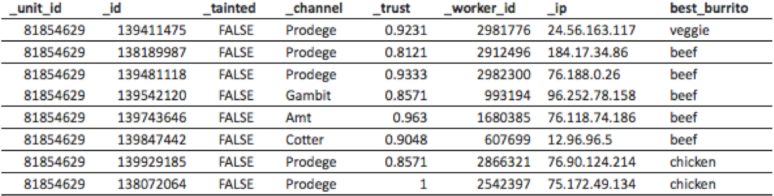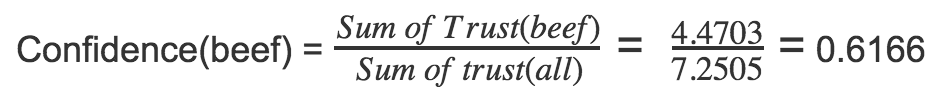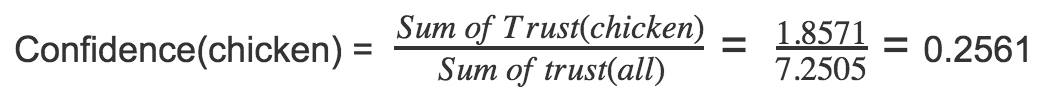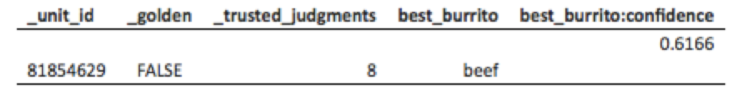Follow

# How to Calculate a Confidence Score

Confidence is a score that accompanies each aggregate (agg) result in an Appen job. It describes the level of agreement between multiple contributors (weighted by the contributors’ trust scores), and indicates our “confidence” in the validity of the result. The aggregate result is chosen based on the response with the greatest confidence.

For example, consider the scenario where a question in a task has three possible answers: “beef,” “chicken,” or “veggie.” The confidence would be calculated in the following three steps:

1. Sum the trust scores of the contributors responsible for each response (this is found in the worker report):

a. Sum of trust(beef) = 4.4703

b. Sum of trust(chicken) = 1.8571

c. Sum of trust(veggie) = 0.9231Fig. 1: A sample full report

2. Sum the trust scores for all responding contributors:

a. Sum of trust(all) = 7.2505

3. Divide each in (1) by (2) to find the confidence score for each response

a.b.c.(Note: All confidence scores on a field sum to 1)

In this case, the aggregate csv for this unit shows that out of eight responses, the beef burrito had the highest confidence score of .6166.Fig. 2: A single result from a sample aggregate report